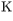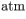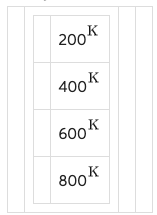# Problem: A tank is filled with an ideal gas at 400  and pressure of 1.00 .Having been heated to 800 , at some point the tank starts to leak. By the time the leak is repaired, the tank is only half full, and the pressure of the remaining gas is again 1.00 . What is the temperature of the gas?

###### FREE Expert Solution

Ideal gas equation:

$\overline{){\mathbf{P}}{\mathbf{V}}{\mathbf{=}}{\mathbf{n}}{\mathbf{R}}{\mathbf{T}}}$

P2 = 2atm

T2 = 800 K

P3 = 1 atm

T3 = ?

The volume of the tank does not change. The gas occupies the tank completely.

98% (208 ratings)###### Problem Details

A tank is filled with an ideal gas at 400and pressure of 1.00.

Having been heated to 800, at some point the tank starts to leak. By the time the leak is repaired, the tank is only half full, and the pressure of the remaining gas is again 1.00. What is the temperature of the gas?